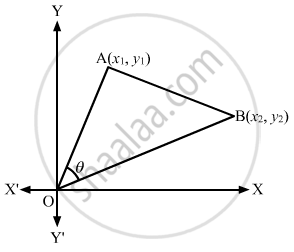Advertisement Remove all ads

# If θ is the Angle Which the Straight Line Joining the Points (X1, Y1) and (X2, Y2) Subtends at the Origin, Prove that - Mathematics

Answer in Brief

If θ is the angle which the straight line joining the points (x1, y1) and (x2, y2) subtends at the origin, prove that  $\tan \theta = \frac{x_2 y_1 - x_1 y_2}{x_1 x_2 + y_1 y_2}\text { and } \cos \theta = \frac{x_1 x_2 + y_1 y_2}{\sqrt{{x_1}^2 + {y_1}^2}\sqrt{{x_2}^2 + {y_2}^2}}$.

Advertisement Remove all ads

#### Solution

Let (x1, y1) and B (x2, y2) be the given points.
Let O be the origin.Slope of OA = m1 = $\frac{y_1}{x_1}$

Slope of OB = m2 = $\frac{y_2}{x_2}$

It is given that $\theta$ is the angle between lines OA and OB.

$\therefore \tan \theta = \frac{m_1 - m_2}{1 + m_1 m_2}$

$= \frac{\frac{y_1}{x_1} - \frac{y_2}{x_2}}{1 + \frac{y_1}{x_1} \times \frac{y_2}{x_2}}$

$\Rightarrow \tan \theta = \frac{x_2 y_1 - x_1 y_2}{x_1 x_2 + y_1 y_2}$

Now,
As we know that

$\cos \theta = \sqrt{\frac{1}{1 + \tan^2 \theta}}$

$\therefore \cos \theta = \frac{x_1 x_2 + y_1 y_2}{\sqrt{\left( x_2 y_1 - x_1 y_2 \right)^2 + \left( x_1 x_2 + y_1 y_2 \right)^2}}$

$\Rightarrow \cos \theta = \frac{x_1 x_2 + y_1 y_2}{\sqrt{{x_2}^2 {y_1}^2 + {x_1}^2 {y_2}^2 + {x_1}^2 {x_2}^2 + {y_1}^2 {y_2}^2}}$

$\Rightarrow \cos \theta = \frac{x_1 x_2 + y_1 y_2}{\sqrt{{x_1}^2 + {y_1}^2} \sqrt{{x_2}^2 + {y_2}^2}}$

Is there an error in this question or solution?
Advertisement Remove all ads

#### APPEARS IN

RD Sharma Class 11 Mathematics Textbook
Chapter 23 The straight lines
Exercise 23.13 | Q 5 | Page 99
Advertisement Remove all ads

#### Video TutorialsVIEW ALL 

Advertisement Remove all ads
Share
Notifications

View all notifications

Forgot password?